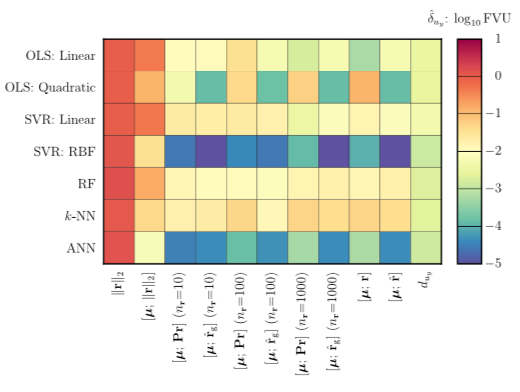# Machine-learning error models for approximate solutions to parameterized systems of nonlinear equations### Abstract

This work proposes a machine-learning framework for constructing statistical models of errors incurred by approximate solutions to parameterized systems of nonlinear equations. These approximate solutions may arise from early termination of an iterative method, a lower-fidelity model, or a projection-based reduced-order model, for example. The proposed statistical model comprises the sum of a deterministic regression-function model and a stochastic noise model. The method constructs the regression-function model by applying regression techniques from machine learning (e.g., support vector regression, artificial neural networks) to map features (i.e., error indicators such as sampled elements of the residual) to a prediction of the approximate-solution error. The method constructs the noise model as a mean-zero Gaussian random variable whose variance is computed as the sample variance of the approximate-solution error on a test set; this variance can be interpreted as the epistemic uncertainty introduced by the approximate solution. This work considers a wide range of feature-engineering methods, data-set-construction techniques, and regression techniques that aim to ensure that (1) the features are cheaply computable, (2) the noise model exhibits low variance (i.e., low epistemic uncertainty introduced), and (3) the regression model generalizes to independent test data. Numerical experiments performed on several computational-mechanics problems and types of approximate solutions demonstrate the ability of the method to generate statistical models of the error that satisfy these criteria and significantly outperform more commonly adopted approaches for error modeling.

Publication
Computer Methods in Applied Mechanics and Engineering, Vol. 348, p.250–296 (2019)